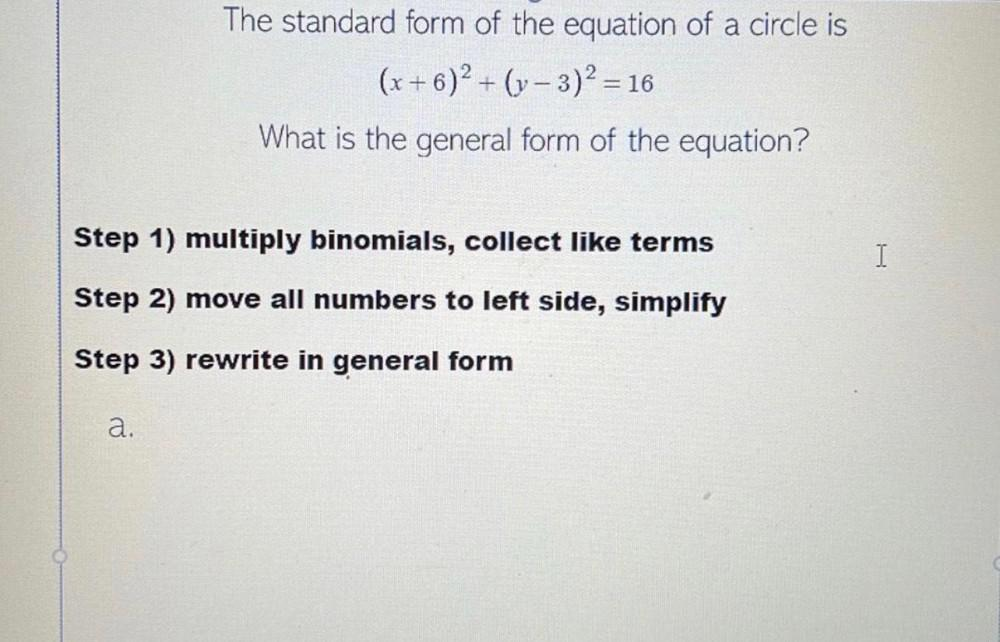Question:

# The standard form of the equation of a circle is (x + 6)2 + (y- 3)2 = 16 What is the general form of the equation? + = Step 1) mThe standard form of the equation of a circle is (x + 6)2 + (y- 3)2 = 16 What is the general form of the equation? + = Step 1) multiply binomials, collect like terms I Step 2) move all numbers to left side, simplify Step 3) rewrite in general form a.